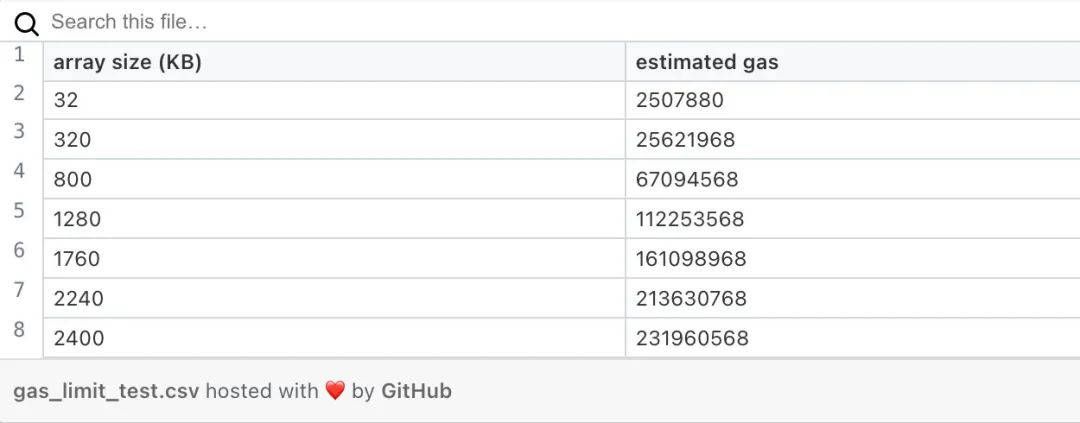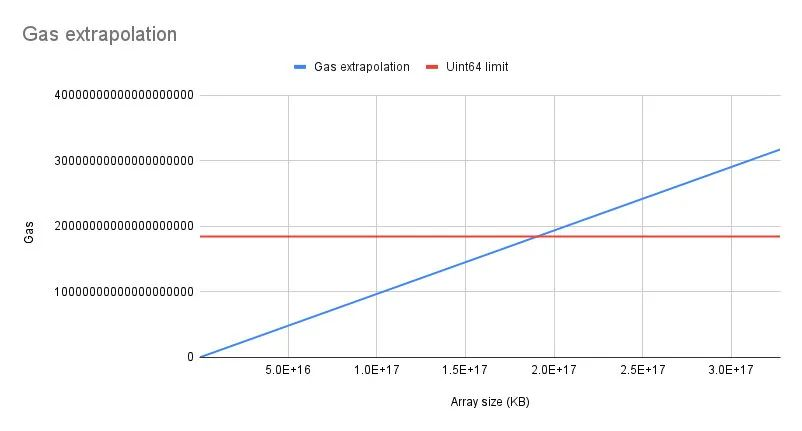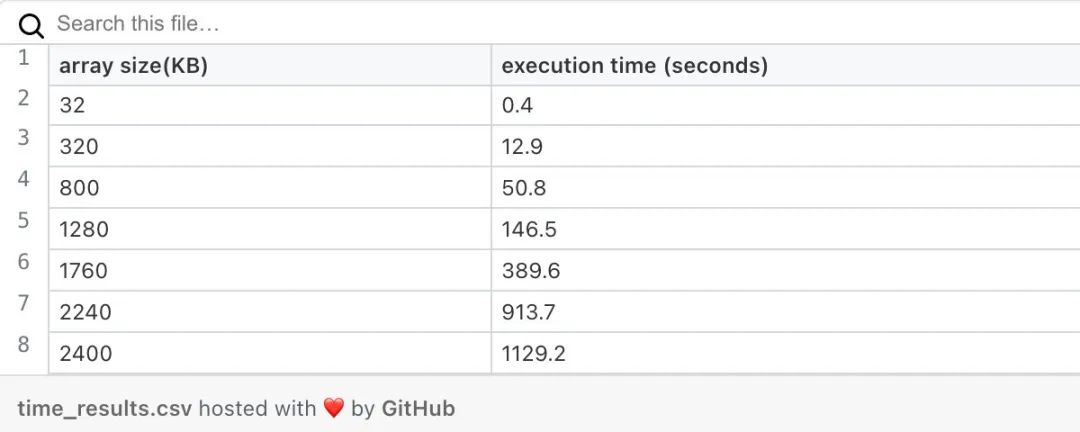# gas限制

``````// SPDX-License-Identifier: MIT
pragma solidity ^0.8.9;

contract ReturnLimitTest {
uint256[] private array;

for (uint256 i = 0; i &lt; count; i++) {
array.push(array.length);
}
}

function getArray() external view returns (uint256[] memory) {
return array;
}
}

import * as hre from "hardhat";
import {program} from "commander";
import {ReturnLimitTest__factory} from "../typechain-types";

async function main() {
program.option("-instance, --instance &lt;string>")
program.parse();

const BN = hre.ethers.BigNumber;
const accounts = await hre.ethers.getSigners();
const factory = new ReturnLimitTest__factory(accounts);
const instance = factory.attach(program.opts().instance);
console.log("array size,estimated gas")
for (let i = 0; i &lt; 10000000000000000; i++) {
const estimatedGas = await instance.estimateGas.getArray({
gasLimit: BN.from("18446744073709551615")
});
console.log((i * 1000).toString() + ',' + estimatedGas.toString());
}
}

main().catch((error) => {
console.error(error);
process.exitCode = 1;
});``````# 执行时间

• CPU - 11代Intel®Core™i7-1165G7 @ 2.80GHz × 8

• Ram - 16GB

• 操作系统- Ubuntu 22.04 LTS

• 磁盘 - 512gb SSD

``````console.log("array size,estimated gas,execution time")
for (let i = 0; i &lt; 10000000000000000; i++) {
const start = Date.now();
const estimatedGas = await instance.estimateGas.getList({
gasLimit: BN.from("18446744073709551615")
});
const finish = Date.now();
console.log((i * 1000).toString() + ',' + estimatedGas.toString() +
',' + (finish - start).toString());
}``````# 分页

• 在数组上定义排序

• 将页码和页面大小添加到API方法(本例中的合约函数)。你的新方法应该返回数组的一部分：array[pagesize:min((page+1)size, array.length)]

• 将数组中元素的总数量添加到响应中，就可以在客户端上找到页面数量。

``````// SPDX-License-Identifier: MIT
pragma solidity ^0.8.9;

contract ReturnLimitTestWithPagination {
uint256[] private array;

for (uint256 i = 0; i &lt; count; i++) {
array.push(array.length);
}
}

function getArray(uint256 page, uint256 pageSize) external view returns (uint256[] memory, uint256) {
require(pageSize > 0, "page size must be positive");
require(page == 0 || page*pageSize &lt;= array.length, "out of bounds");
uint256 actualSize = pageSize;
if ((page+1)*pageSize > array.length) {
actualSize = array.length - page*pageSize;
}
uint256[] memory res = new uint256[](actualSize);
for (uint256 i = 0; i &lt; actualSize; i++) {
res[i] = array[page*pageSize+i];
}
return (res, array.length);
}
}``````

``````import * as hre from "hardhat";
import {program} from "commander";
import {ReturnLimitTestWithPagination__factory} from "../typechain-types";

async function main() {
program.option("-instance, --instance &lt;string>")
program.parse();

const accounts = await hre.ethers.getSigners();
const factory = new ReturnLimitTestWithPagination__factory(accounts);
const instance = factory.attach(program.opts().instance);
console.log("array size,execution time,one call execution times")
for (let i = 1; i &lt; 10000000000000000; i++) {
const start = Date.now();
const oneCall = [];
for (let j = 0; j &lt; i; j++) {
const start = Date.now();
await instance.estimateGas.getArray(j, 1024);
oneCall.push((Date.now() - start));
}
const finish = Date.now();
console.log((i * 1024 * 32).toString() + ',' +
(finish - start).toString() + ',' + oneCall.join(";"));
}
}

main().catch((error) => {
console.error(error);
process.exitCode = 1;
});````````````await instance.getArray(0, 1024, {
blockTag: 1234,  // block number, hash, or tag (eg. latest)
});``````

# 结论

• 返回大数组的函数的主要问题是调用执行时间。

• 通过分页，我们可以使调用时间变得合理，并可以获取部分数据。如果应用程序的for逻辑可以同时处理数组的一部分，就可以使用该模式。